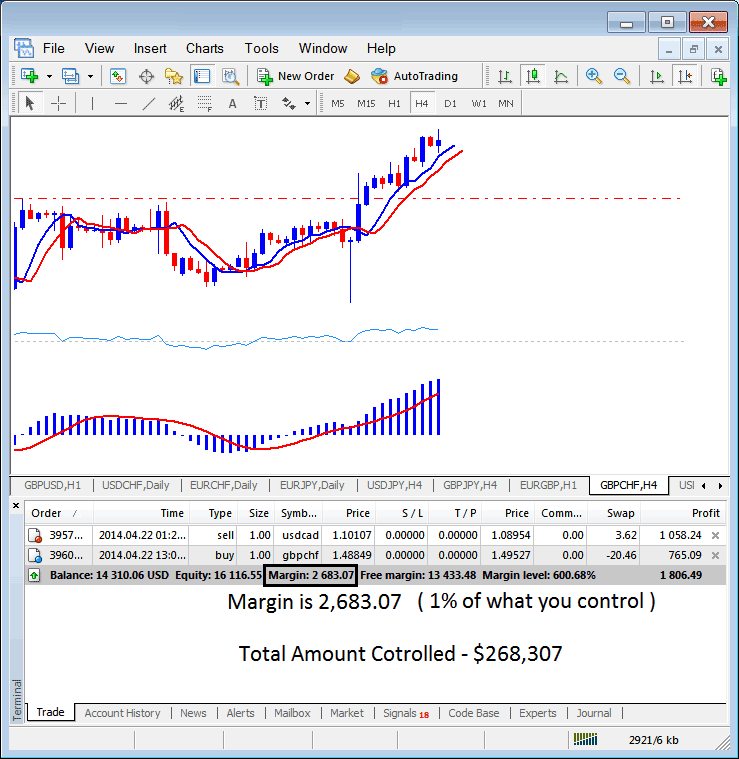# What is The Difference Between Maximum Indices Trading Leverage and Used Indices Trading Leverage?

You should note that there is a difference between maximum indices trading leverage ( indices trading leverage given by your broker which is the highest stock indices leverage you can trade with if you choose to) and used indices trading leverage ( indices trading leverage depending on the lots you have opened/open positions). One is the broker's (Maximum) and the other is trader's (Used). To explain this concept we shall use the stock indexes trading example above:

If your indices broker has given you 100:1 Maximum stock indexes trading leverage, but you only open 1 lot of 100,000 dollars then Used indices trading leverage is:

100,000 dollars (1 lot ): 10,000 dollars (your money)

The example explained and illustrated below, the set stock indices leverage is 100:1, the margin which is 1% is \$2683.07, therefore the total amount controlled by the indices trader is: \$268,307 - this is because with this indices trading leverage the indices trader has used little of his money and borrowed the rest, with this set at 100:1, the indices trader is using 1 % of their capital, this 1% is \$2683.07, if 1% is \$2683.07 then 100% is \$268,307What is The Difference Between Maximum Indices Trading Leverage and Used Indices Trading Leverage?

In the above image example, the indices trader is using \$2683.07, the total controlled amount is \$268,307, but account equity is 16,116.55, therefore used stock indices leverage is (\$268,307 divide by 16,116.55) = 16.64 : 1

16.64 : 1

In the indices trading account above even though the maximum indices trading leverage set stock indices leverage is 100:1, Used indices trading leverage is 16.64 : 1

Start Trading Crypto CFDs with a \$30 Bonus